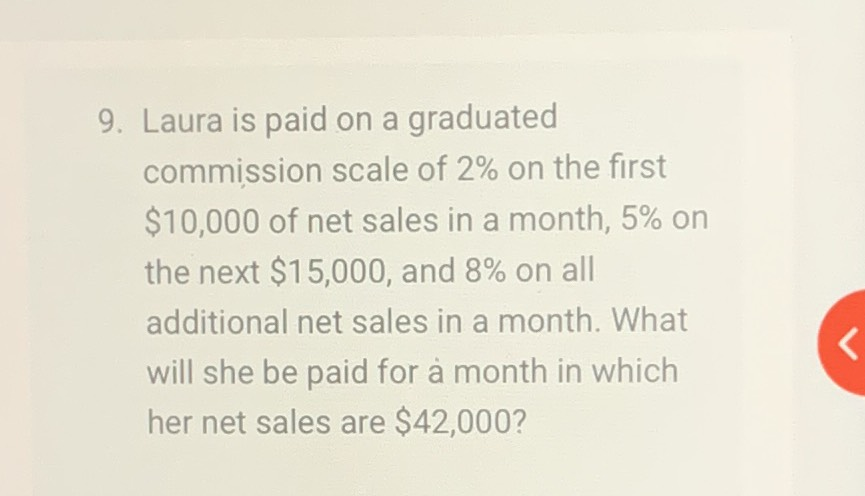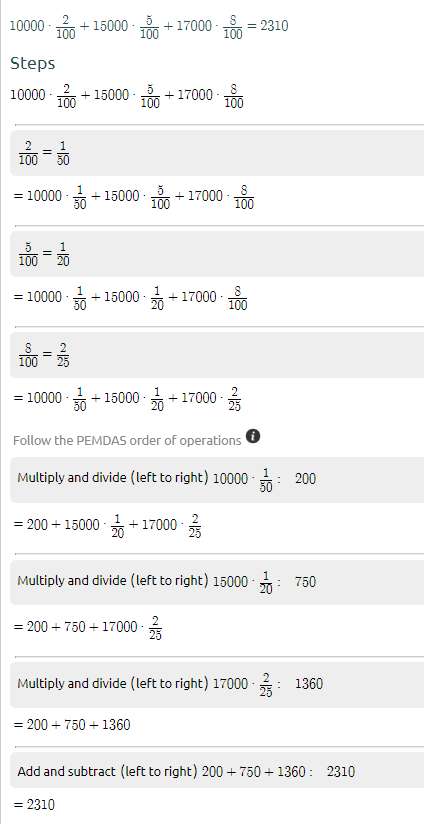### Still have math questions?

Arithmetic
Question9. Laura is paid on a graduated commission scale of $$2 \%$$ on the first

$$\ 10,000$$ of net sales in a month, $$5 \%$$ on the next $$\ 15,000$$ , and $$8 \%$$ on all additional net sales in a month. What will she be paid for a month in which her net sales are $$\ 42,000$$ ?